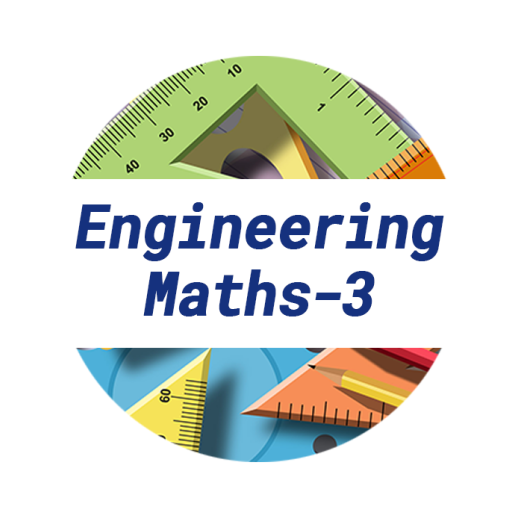#### Current Version : V1.3 Downloads : 28,235 Size : 7.7 MB

Engineering Maths 3 is an app that can be used by Engineering students for quick referral of the formulas during examination time. The app is very much useful for engineering students studying in 2nd year of Engineering of various Universities like Gujarat Technological University (GTU), Saurashtra University, Gujarat University, etc., or IITs or NITs. The app has simple and easy to use UI for quick reference of the basic maths integration formula or differentiation formula. The app shows the necessary engineering mathematics formulas with equations and diagram for detail explanation. You can even share a screenshot of the formula with your friends using various messaging app available on your phone.

<b>Formula covered in the app are</b>:

1) <b>Special Function</b>
- Beta Function
- Gamma Function
- Error Function
- Complementary Error Function
- Unit Step Function
- Pulse of Unit Height
- Sinusoidal Pulse Function
- Rectangle Function
- Gate Function
- Dirac Delta Function
- Square Wave Function
- Saw Tooth Wave Function
- Triangular Wave Function
- Half/Full Rectified Sine Wave Function
- Bessel's Function

2) <b>Fourier Series</b>
- Fourier Series Interval (C, C+2l)
- Leibnitz's Formula
- Factoring Formula
- Fourier Series Interval (0, 2l), (0, 2π), (-l,l), (-π,π)
- Fourier Series for Odd & Even Function
- Half Range Series
- Fourier Transform

3) <b>Differential Equation</b>
- Differential Equation
- Ordinary Differential Equation
- Partial Differential Equation
- Order of Differential Equation
- The degree of Differential Equation
- Linearity of Differential Equation
- Exact Differential Equation
- Non-Exact Differential Equation
- Orthogonal Trajectory
- Higher Order Linear Differential Equation with constant co-efficient
- Auxiliary Equation and second order differential equation
- Method for Finding C.F. of Higher Order Differential Equation
- Method of finding the particular integral
- Method of Undetermined Co-efficient
- Definition: Wronskian
- Method of Variation of Parameters
- Cauchy - Euler Equation
- The solution of Differential Equation by One of Its Solution

4) <b>Series Solution of Differential Equation</b>
- Power Series
- Analytic Function
- Ordinary and Singular Point
- Regular/Irregular Singular Point
- Power Series Solution at an Ordinary Point
- Frobenius Method

5) <b>Laplace Transform</b>
- Properties of Laplace Transforms
- Laplace Transform Table
- Laplace Transform of some Standard Function
- Theorem: First Shifting Theorem
- Theorem: Differentiation of Laplace Transform
- Theorem: Integration of Laplace Transform
- Theorem: Laplace Transform of integration of a function
- Laplace Transform of Unit Step Function
- Theorem: Second Shifting Theorem
- First Shifting Theorem
- Partial Fraction Method
- Second Shifting Theorem
- Inverse Laplace Transform of Derivatives
- Convolution Product
- Theorem: Convolution Theorem
- Theorem: Derivative of Laplace Transform

6) <b>Partial Differential Equation (PDE)</b>
- Partial Differential Equation
- Order/Degree of Differential Equation
- Formation of Partial Differential Equation
- Lagrange's Differential Equation
- Non-Linear Partial Differential Equation of First Orde
- Charpit's Method
- Method of Separation of Variables
- Classification of Second Order Partial Differential Equation

--------------------------------------------------------------------------------------------------
This App is developed at ASWDC by Twisha Kotecha (150540107049), a 6th Sem CE Student. ASWDC is Apps, Software, and Website Development Center @ Darshan Institute of Engineering & Technology, Rajkot run by Students & Staff of Computer Engineering Department

Call us: +91-97277-47317

Write to us: aswdc@darshan.ac.in
Visit: http://www.aswdc.in http://www.darshan.ac.in

ASWDC is established by Department of Computer Engineering where students work on live projects under guidance of staff and industry experts. Students are getting extensive knowledge and industrial experience of cutting edge technologies. ASWDC fills gap between academic curriculum and industry expectation.